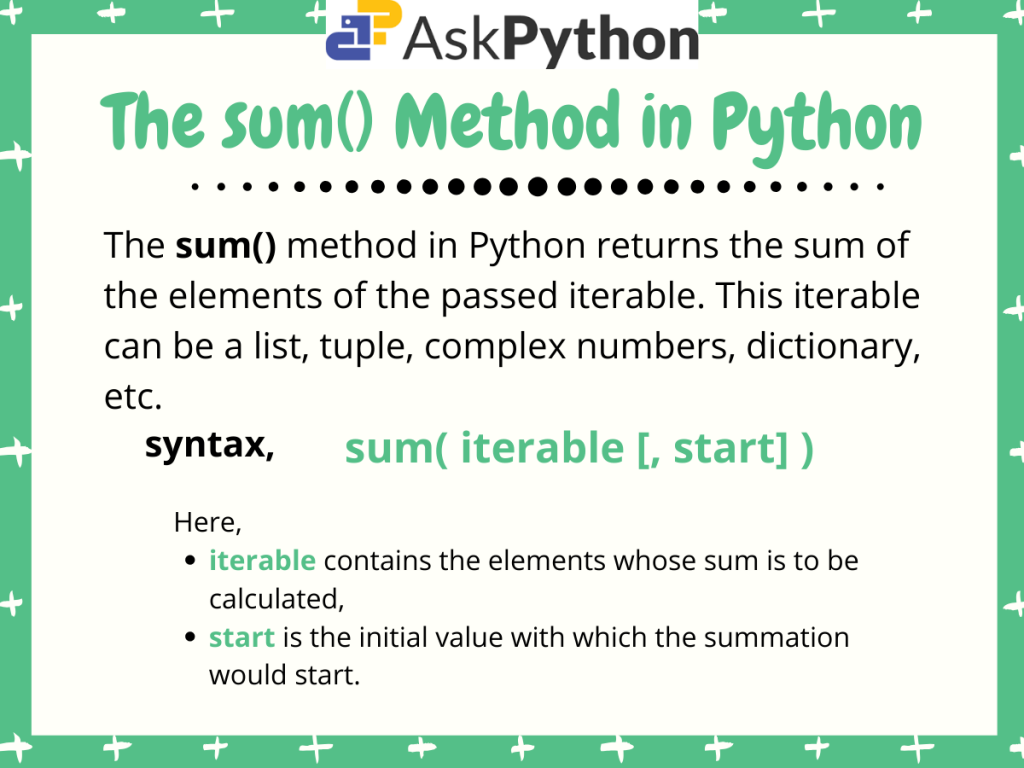# How to Use The Python sum() Function## Introduction

In this tutorial, we are going to discuss the Python sum() method.

The Python `sum()` method is a built-in method that returns the summation of all the elements of the passed iterable.

## The Python sum() function

Let us look at the syntax for using the sum() method in Python.

```sum(iterable[, start])
```

Here,

• iterable can be any iterable object containing the values for which we need to calculate the sum. It can be a list, tuple, or dictionary,
• start is the initial value with which the addition is going to take place. By default, the start value is set to 0.

Now let us look at some examples to have a clear understanding of the method’s use and working.

## Using sum() for a list, tuple, complex numbers, floats, etc.

As mentioned earlier, the `sum()` method can calculate the sum of values passed in the form of list, tuple or dictionary that is, any iterable. But the condition is, the iterable should contain some value, or else, an error is raised.

Let us familiarize ourselves with the method by finding the sum of elements of a list of integers, tuple, dictionary and a list of complex numbers.

```#using built-in sum() in Python

list1=[1,2,3,4,5]
tuple1=(10,20,30)
dict1={ 0:11, 1:22, 2:33, 3:44 }
complx_nums=[ 4+3j, 7+5j, 8+3j]

print("sum of list elements:",sum(list1,5))
print("sum of tuple elements:", sum(tuple1))
print("sum of dictionary keys:", sum(dict1))
print("sum of complex numbers:", sum(complx_nums))
```

Output:

```sum of list elements: 20
sum of tuple elements: 60
sum of dictionary keys: 6
sum of complex numbers: (19+11j)
```

Here,

• We firstly initialize list1, tuple1, dict1, and complx_num with some values,
• Then we simply pass these iterable to the `sum()` method individually,
• For calculating the sum of list1 elements we set a start value of 5 and for the rest, we do not pass any start parameter(by default set to 0).

Hence, we get the desired output. For every case, we get the sum of the respective iterable elements.

## Python sum() vs NumPy sum()

The NumPy module in Python comes with a `sum()` method defined in it. It is used to find the sum of NumPy array elements. But, this method can also find the sum of elements for any other iterable in python containing some values.

Let’s compare the output for both `sum()` and `numpy.sum()` in Python for some iterable objects.

```#numpy sum() vs python sum()

import numpy as np

list1=[1,2,3,4,5]
np_arr = np.array([1,2,3,4,5])

print("Built-in sum() result:",sum(list1))
print("numpy sum() for np array:",np.sum(np_arr))
print("numpy sum() for list:",np.sum(list1))
```

Output:

```Built-in sum() result: 15
numpy sum() for np array: 15
numpy sum() for list: 15
```

As we can see, both the `sum()` and `np.sum()` methods return the same result for the list list1.

For calculating the sum of iterable objects like lists, tuples and dictionaries, the built-in `sum()` method is much faster and easy to use than the numpy’s `sum()` method.

But when it comes to numpy arrays, the `np.sum()` method appears to be faster and reliable. This is simply because NumPy uses a vectorized approach.

## Conclusion

Hence, in this tutorial, we learned about the built-in `sum()` method in Python as well as a comparison between Python sum() and numpy.sum() methods. I hope you now have a clear understanding of the method.

For any further questions feel free to common below.